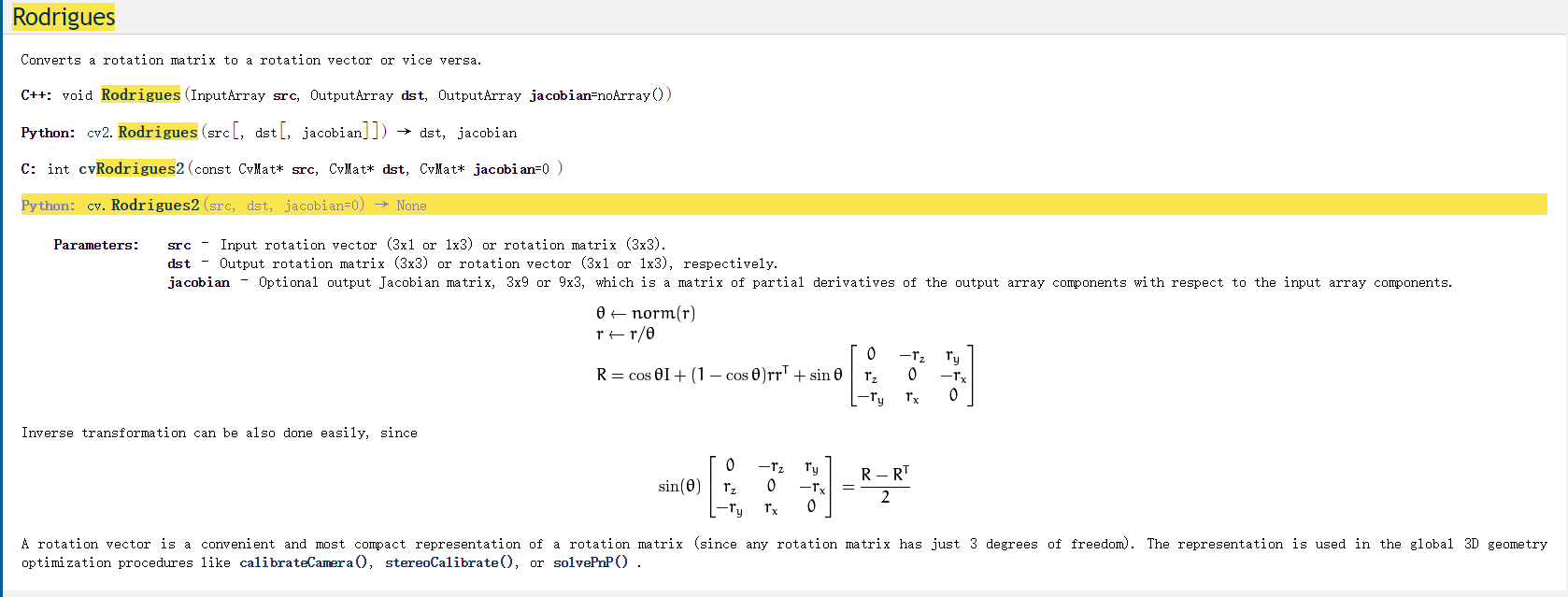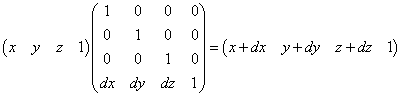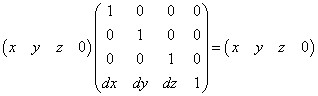罗德里格斯变换

当处理三维空间的时候，常常需要用3X3矩阵表征空间旋转。
这种表示方法通常是最方便的，因为一个向量乘以该矩阵等价于该向量某种方式的旋转。不便之处是它不能直观显示3X3矩阵的旋转含义。
另外一个容易可视化的表示方式是用向量形式表示旋转，而该旋转每次用单个角度来操作。这种情况下，最标准的方式是仅用一个向量来说明绕坐标轴的旋转，向量长度表示绕轴逆时针旋转的角度。这个很容易实现，由于方向可以用任意长度的向量表示，因此我们选择向量的长度表示旋转角度。两种表述方式的关系是矩阵和向量可以用罗德里格斯变换关联。
设r为三维向量r=[rx,ry,rz]，这个向量含蓄地定义 ，旋转量用r的长度表示。我们能够将这种以坐标轴-标量形式表示的旋转转换为一个旋转矩阵R：

$R=cos\left(\theta \right).I+\left(1-cos\left(\theta \right)\right).r{r}^{T}+sin\left(\theta \right).\left[\begin{array}{ccc}0& -{r}_{z}& {r}_{y}\\ {r}_{z}& 0& -{r}_{x}\\ -{r}_{y}& {r}_{x}& 0\end{array}\right]$

我们也能反向从坐标轴表现形式得到旋转矩阵：

$sin\left(\theta \right).\left[\begin{array}{ccc}0& -{r}_{z}& {r}_{y}\\ {r}_{z}& 0& -{r}_{x}\\ {r}_{y}& {r}_{x}& 0\end{array}\right]=\frac{R-{R}^{T}}{2}$

因此我们发现一种表示形式（矩阵表示）更方便地计算，而另一种表现形式（罗德里格斯）更易于理解。OpenCV为我们提供了相互转换的函数：假定我们有向量r和对应的旋转矩阵R，设置src为3X1向量r,dst为3x3旋转矩阵R。相反，我们可以设置src为3x3旋转矩阵R，以及dst为3X1向量r.不论在哪种情况下，函数Rodrigues()都会正确执行。最后的参数是可选的。如果jacobian不为NULL，那么它应该是一个3X9或9x3矩阵的指针，其元素是对应输入数组元素的输出数组元素的偏微分。Jacobian输出主要用于函数cvFindExtrinsicCameraParameters()和cvCalibrateCamera2()的输出从罗德里格斯的1x3或3x1坐标轴角度向量格式转换为旋转矩阵。如果是这样，请将jacobian设置为NULL。
以下是之前使用过的程序代码，里面有使用到罗德里格斯变换。

  void project2Ellipse(double &radius, double &Rx, double &Ry, double &Rz, double &Tz, int &sigma, vector<Point2f> &imgCenter, Mat &img)
{
/////STEP-1:设定相机模型
vector<Point2f>  in_pnts;
vector<Point3f>  out_pnts;
static vector<Point2f>  boundaryPnts;
/////STEP-1.1:设定相机内参数；
double fx = 5769.518128499;
double fy = 5769.304247163;
/*double cx = 2575.315753418;
double cy = 1695.067332223;*/
double cx = 335;
double cy = 255;
Mat Camera_Matrix = (Mat_<double>(3, 3) << fx, 0, cx, 0, fy, cy, 0, 0, 1);
/////STEP-1.2：设定相机畸变参数
/*double k1 = -0.124141797;
double k2 = 0.197321413;
double k3 = -0.000768697;
double k4 = -0.001034843;*/
double k1 = 0;
double k2 = 0;
double k3 = 0;
double k4 = 0;
vector<double> Distort_Coefficients;
Distort_Coefficients.push_back(k1);
Distort_Coefficients.push_back(k2);
Distort_Coefficients.push_back(k3);
Distort_Coefficients.push_back(k4);
/////STEP-1.3:设定相机外参数：R，T（旋转、平移）
//double Tx = -189.425316;
//double Ty = -156.421414;
double Tx = 0;
double Ty = 0;
Mat NewProMatrix = (Mat_<double>(3, 1) << Tx, Ty, Tz);
Mat RectTransformation = (Mat_<double>(3, 1) << Rx, Ry, Rz);
/////STEP-1.4:设定图像大小
int length = 3456;
int width = 5184;
Mat srcImg = Mat::Mat(length, width, CV_8UC1, Scalar(0)) + 50;

/////STEP-2:使用 projectPoints 把理论圆心点影射到图像上，计算得到椭圆上的理论圆心。
Mat _RectTransformation;
RectTransformation.copyTo(_RectTransformation);
if (RectTransformation.cols == 1 || RectTransformation.rows == 1)
{
Rodrigues(RectTransformation, _RectTransformation);
}
Point3f centerPnt = Point3f(0, 0, 0);
vector<Point3f> centerPoint;
centerPoint.push_back(centerPnt);
projectPoints(centerPoint, _RectTransformation, NewProMatrix,
Camera_Matrix, Distort_Coefficients, imgCenter);
////STEP-1.5:遍历图像中的每一个像素

for (int i = 0; i < srcImg.rows; i++)
{
for (int k = 0; k < srcImg.cols; k++)
{
in_pnts.push_back(Point2f(k, i));
}
}
/////STEP-3:使用函数project2CalibrationBoard将图像的任意一个像素影射到标定板上
CoreAlgorithm::project2CalibrationBoard(in_pnts, out_pnts,
Camera_Matrix, Distort_Coefficients, RectTransformation, NewProMatrix);
/////STEP-4:根据标定板上的理论圆，计算该影射点是否在该圆内。
/////若在，则为白色，否则为黑色。  采用到原点距离的方法设定，严格小于设定方法
for (int i = 0; i < out_pnts.size(); i++)
{
double Dis = sqrt(pow((out_pnts[i].x - centerPnt.x), 2) + pow((out_pnts[i].y - centerPnt.y), 2) + pow((out_pnts[i].z - centerPnt.z), 2));
{
srcImg.at<uchar>(in_pnts[i].y, in_pnts[i].x) = 200;
}
else
{
srcImg.at<uchar>(in_pnts[i].y, in_pnts[i].x) = 50;
}
}
}



附：

///求出标定板特征点的三维点平面点
///@param R 3x1的旋转向量 或3x3的旋转矩阵
void  project2CalibrationBoard(const vector<Point2f>& src, vector<Point3f>& pnts3d,
const Mat& cameraMatrix, vector<double> distCoeffs, const Mat& R, const Mat& T)
{
vector<Point2f> pnts2d;
Mat _R;
R.copyTo(_R);
if (R.cols == 1 || R.rows == 1)
{
Rodrigues(R, _R);
}
T.col(0).copyTo(_R.col(2));
invert(_R, _R);///////求逆矩阵
undistortPoints(src, pnts2d, cameraMatrix, distCoeffs, _R);
for (unsigned int i = 0; i < pnts2d.size(); i++)
{
pnts3d.push_back(Point3f(pnts2d[i].x, pnts2d[i].y, 0));
}
}

文章进行到这里，你可能会产生一个疑问，罗德里格斯变换只是对于普通的3x1矩阵或3x3矩阵，那图形学中为什么常常会使用齐次坐标变化呢？这也是我自己心中的一个疑惑。

4D向量是由3D坐标(x,y,z)和齐次坐标w组成，写作(x,y,z,w)。
在3D世界中为什么需要3D的齐次坐标呢？简单地说明一下，在一维空间中的一条线段上取一点x，然后我们想转移x的位置，那我们应该是x’=x+k，但我们能使用一维的矩阵来表示这变换吗？不能，因为此时一维的矩阵只能让x点伸缩。但如果变成了一维的齐次空间[k 1]就很容易地做到。同样地，在二维空间中，某一图形如果不使用二维的齐次坐标，则只能旋转和伸缩，却不能平移。
因此，我们在3D坐标中使用齐次坐标，是为了物体在矩阵变换中，除了伸缩旋转，还能够平移，如下运算：既然了解了使用齐次坐标的意义，我们下一步就要了解一下齐次坐标w是什么意义。设w=1，此时相当于我们把3D的坐标平移搬去了w=1的平面上，4D空间的点投影到w=1平面上，齐次坐标映射的3D坐标是（x/w,y/w,z/w），也就是（x,y,z）。（x,y,z）在齐次空间中有无数多个点与之对应。所有点的形式是（kx,ky,kz,k），其轨迹是通过齐次空间原点的“直线”（其实每个点相当于3D的坐标世界）。此时不能平移变换了。这个现象是非常有用的，因为有些向量代表“位置”，应当平移，而有些向量代表“方向”，如表面的法向量，不应该平移。从几何意义上说，能将第一类数据当作”点”，第二类数据当作”向量”。可以通过设置w的值来控制向量的意义。
简单点来说，齐次矩阵，增加了一个维度，就能表示平移了。把所有变换都表示到一个矩阵中，方便进行矩阵连乘。